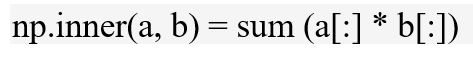# numpy.inner() in python

`numpy.inner(arr1, arr2)`: Computes the inner product of two arrays.```Parameters :
arr1, arr2 : array to be evaluated.

Return:  Inner product of the two arrays.```

Code #1 :

 `# Python Program illustrating  ` `# numpy.inner() method  ` ` `  `import` `numpy as geek  ` ` `  `# Scalars  ` `product ``=` `geek.inner(``5``, ``4``)  ` `print``(``"inner Product of scalar values : "``, product)  ` ` `  `# 1D array  ` `vector_a ``=` `2` `+` `3j` `vector_b ``=` `4` `+` `5j` ` `  `product ``=` `geek.inner(vector_a, vector_b)  ` `print``(``"inner Product : "``, product)  `

Output:

```inner Product of scalar values :  20
inner Product :  (-7+22j)
```

Code #2 : As normal matrix multiplication

 `# Python Program illustrating  ` `# numpy.inner() method  ` ` `  `import` `numpy as geek  ` ` `  `# 1D array  ` `vector_a ``=` `geek.array([[``1``, ``4``], [``5``, ``6``]])  ` `vector_b ``=` `geek.array([[``2``, ``4``], [``5``, ``2``]])  ` ` `  `product ``=` `geek.inner(vector_a, vector_b)  ` `print``(``"inner Product : \n"``, product)  ` ` `  `product ``=` `geek.inner(vector_b, vector_a)  ` `print``(``"\ninner Product : \n"``, product)  `

Output:

```inner Product :
[[18 13]
[34 37]]

inner Product :
[[18 34]
[13 37]]
```

My Personal Notes arrow_drop_upAspire to Inspire before I expire

If you like GeeksforGeeks and would like to contribute, you can also write an article using contribute.geeksforgeeks.org or mail your article to contribute@geeksforgeeks.org. See your article appearing on the GeeksforGeeks main page and help other Geeks.

Please Improve this article if you find anything incorrect by clicking on the "Improve Article" button below.

Article Tags :

Be the First to upvote.

Please write to us at contribute@geeksforgeeks.org to report any issue with the above content.